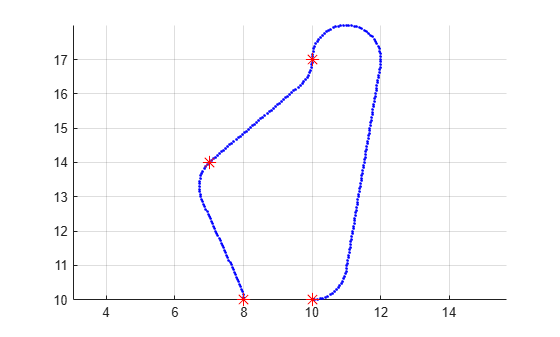Main Content

# interpolate

Interpolate points along path

## Syntax

``interpolate(path,numStates)``

## Description

example

````interpolate(path,numStates)` inserts a number of states in the path and ensures the distribution of all the points in the path to be uniform. The function preserves all the existing states in the path. The value of `numStates` must be greater than or equal to the number of existing states in the path.```

## Examples

collapse all

Create a navPath object based on multiple waypoints in a Dubins space.

`dubinsSpace = stateSpaceDubins([0 25; 0 25; -pi pi])`
```dubinsSpace = stateSpaceDubins with properties: SE2 Properties Name: 'SE2 Dubins' StateBounds: [3×2 double] NumStateVariables: 3 Dubins Vehicle Properties MinTurningRadius: 1 ```
`pathobj = navPath(dubinsSpace)`
```pathobj = navPath with properties: StateSpace: [1×1 stateSpaceDubins] States: [0×3 double] NumStates: 0 ```
```waypoints = [... 8 10 pi/2; 10 12 pi/4; 12 17 pi/2; 11 10 -pi]; append(pathobj, waypoints);```

Interpolate that path so that it contains exactly 250 points.

`interpolate(pathobj, 250)`

Visualize the interpolated path and the original waypoints.

```figure; grid on; axis equal; hold on; plot(pathobj.States(:,1), pathobj.States(:,2), ".b"); plot(waypoints(:,1), waypoints(:,2), "*r", "MarkerSize", 10)```Calculate length of path.

```len = pathLength(pathobj); disp("Path length = " + num2str(len))```
```Path length = 19.37 ```

## Input Arguments

collapse all

Path object, specified as a `navPath` object.

Data Types: `object`

Number of states inserted in the path, specified as a nonnegative integer. Its value must be greater than or equal to the number of existing states in the path.

Data Types: `double`

## See Also

Introduced in R2019b